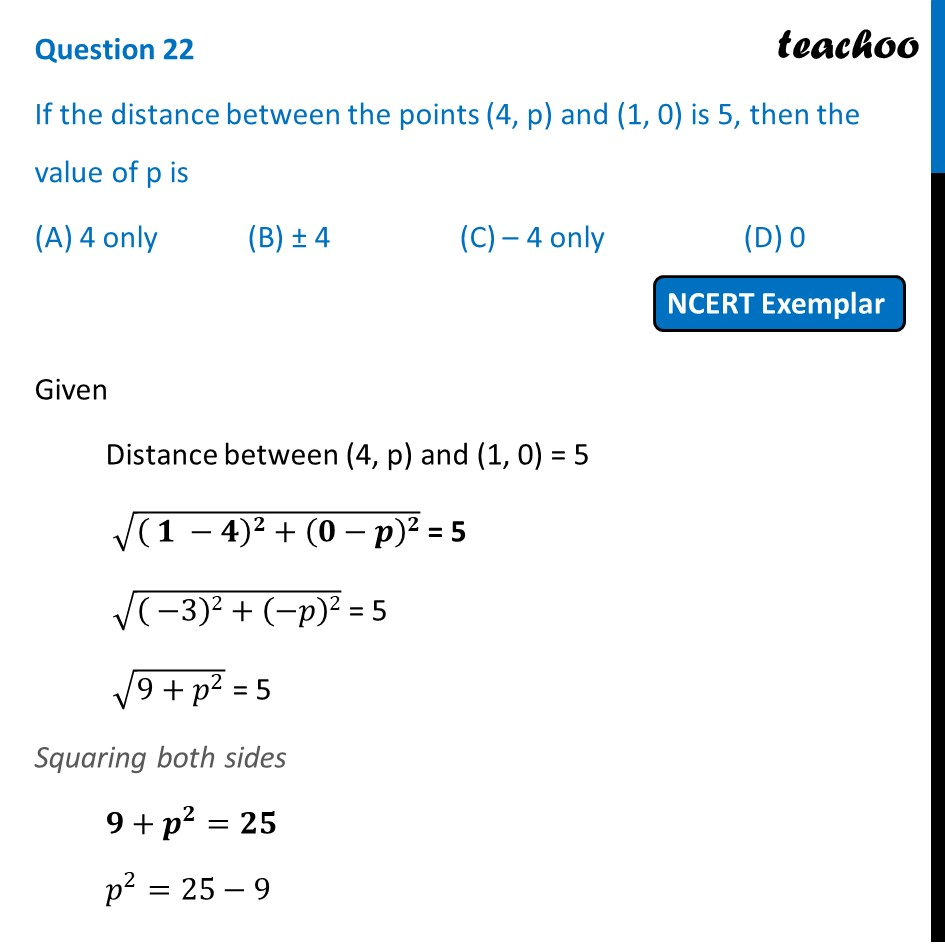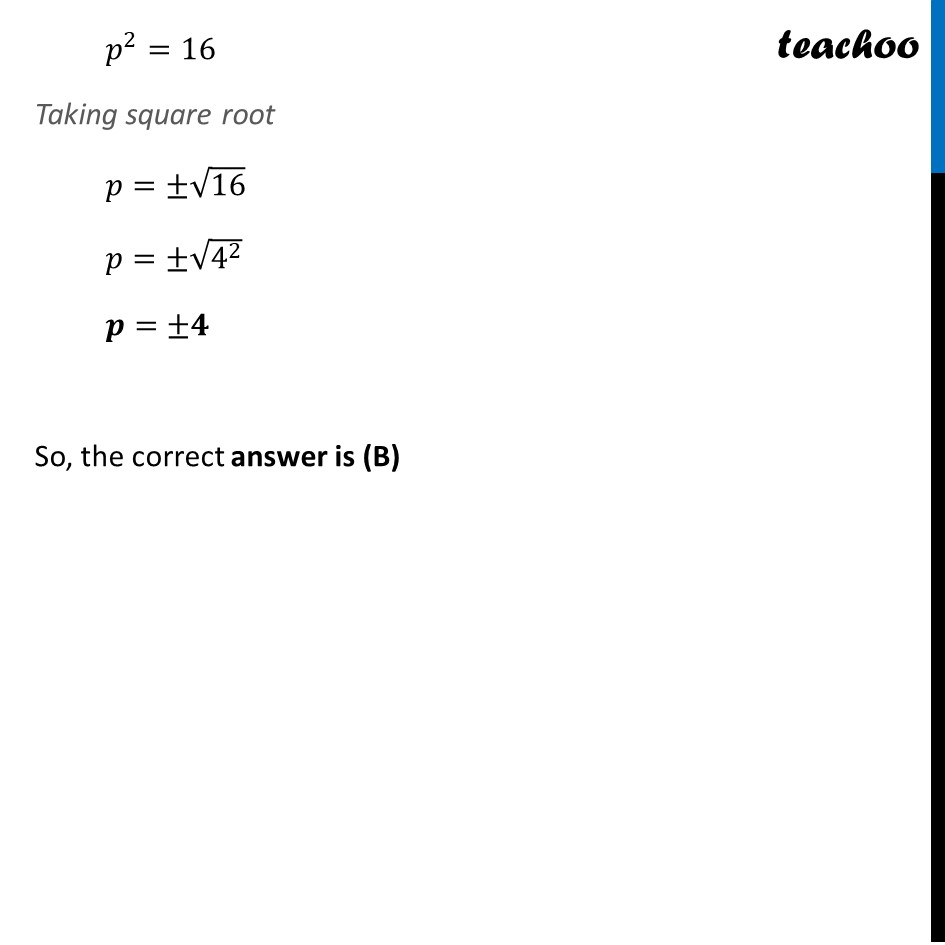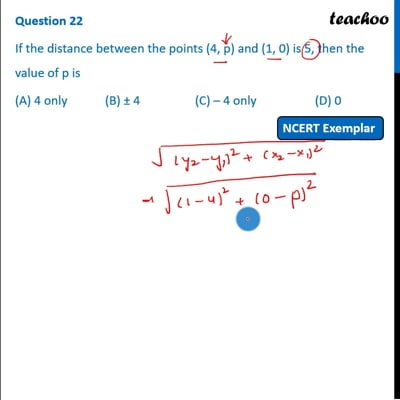NCERT Exemplar - MCQ

Chapter 7 Class 10 Coordinate Geometry
Serial order wise

## (D) 0This video is only available for Teachoo black users

Introducing your new favourite teacher - Teachoo Black, at only ₹83 per month

### Transcript

Question 22 If the distance between the points (4, p) and (1, 0) is 5, then the value of p is (A) 4 only (B) ± 4 (C) – 4 only (D) 0 Given Distance between (4, p) and (1, 0) = 5 √(( 𝟏 −𝟒)𝟐+(𝟎−𝒑)𝟐) = 5 √(( −3)2+(−𝑝)2) = 5 √(9+𝑝^2 ) = 5 Squaring both sides 𝟗+𝒑^𝟐=𝟐𝟓 𝑝^2=25−9 𝑝^2=16 Taking square root 𝑝=±√16 𝑝=±√(4^2 ) 𝒑=±𝟒 So, the correct answer is (B)